### Maximum Flow Problems

The maximum flow problem (MFP) can be stated as follows: Given a directed graph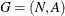with capacity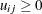on each arc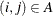, a source nodeand a sink node, find the maximum flow that can go fromto, while obeying the flow conservation constraints at each node. You can solve such problems using the MAXFLOW option in the call to PROC NETFLOW.

Ordinarily many, if not all, arcs in an MFP network have capacities, and it is common that these arcs have zero costs. However, the NETFLOW procedure enables you to have nonzero costs to influence the optimal solution in cases where multiple maximum flow patterns are known to exist.

The following two subsections explain the role of the EXCESS= option in solving pure and generalized maximum flow problems.

#### The EXCESS=ARCS Option

Consider a maximum flow problem involving a pure network. Assume that you do not explicitly specify the EXCESS= option (the EXCESS=ARCS option is used by the procedure by default). The NETFLOW procedure sets up the problem in the following manner:

1. The source node is assigned a supdem value equal to INFINITY1.

2. The sink node is assigned a supdem value equal to(INFINITY1).

3. If there is no existing arc between the source node and the sink node, an arc called the bypass arc directed from the source node to the sink node is added.

4. If there is an existing arc between the source node and the sink node, a dummy node is used to break up what would have been a single bypass arc: source —> sink gets transformed into two arcs, source —> dummy —> sink.

5. If you are maximizing, then the cost of the bypass arc(s) is equal to1 if all other arcs have zero costs; otherwise the cost of the bypass arc(s) is equal to(INFINITY / BYPASSDIV).

6. If you are minimizing, then the cost of the bypass arc(s) is equal to 1 if all other arcs have zero costs; otherwise the cost of the bypass arc(s) is equal to INFINITY / BYPASSDIV.

You can specify the value of the INFINITY= option in the procedure statement, or you can use the default value of 99999999. You can also specify the BYPASSDIV= option. The default value for the BYPASSDIV= option is 100.

This scenario is depicted in Figure 6.29. Since the cost of the bypass arc is unattractive, the optimization process minimizes the flow through it, thereby maximizing the flow through the real network. See the first subsection in Example 6.10 for an illustration.

Figure 6.29: Pure Maximum Flow Problem, EXCESS=ARCS Option Specified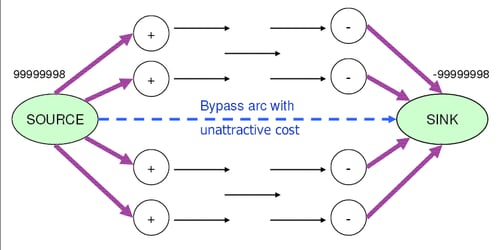This method of setting up a maximum flow problem does come with a drawback. It is likely to produce incorrect results if the following occur:

• the maximum flow is greater than INFINITY1, or

• the cost of the bypass arc is insufficiently unattractive to ensure that the entire flow traverses the real network and not through the bypass arc

Additionally, numbers of large magnitude can cause problems during optimization, including numerical instability and loss of precision. In the next section, we explain how to overcome these difficulties when solving maximum flow problems.

#### The EXCESS=SLACKS Option

Assume you have a pure maximum flow problem and you specify the EXCESS=SLACKS option. The NETFLOW procedure sets up the problem in the following manner:

• The source node is assigned a missing S supply value.

• The sink node is assigned a missing D demand value.

Since this network contains a node with a missing S supply value and a node with a missing D demand value, we have a situation similar to the one described in the section Handling Missing Supply and Demand Simultaneously. Both of these nodes have slack variables. Usually, slack variables have zero objective function coefficients, but because the MAXFLOW option is specified, one of the slack variables must be attractive enough to make it worthwhile for flow to traverse the network. Figure 6.30 presents the scenario clearly.

If you are maximizing, then the objective function coefficient of the slack variable associated with the sink node is1 if all other arcs have zero costs. Otherwise it is(INFINITY / BYPASSDIV). If you are minimizing, then the objective function coefficient of the slack variable associated with the sink node is 1 if all arcs have zero costs. Otherwise it is INFINITY / BYPASSDIV. See the second subsection in Example 6.10 for an illustration of the EXCESS=SLACKS option in pure maximum flow problems.

Note: If the MAXFLOW option is not specified, these slack variables assume zero objective function coefficients, and the MFP may not be solved properly.

Figure 6.30: Pure Maximum Flow Problem with EXCESS=SLACKS Option Specified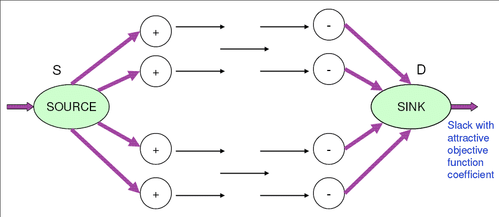When you use the MAXFLOW option, the procedure sets up the problem in such a way that maximum flow traverses the network. This enables you to transform certain types of problems into maximum flow problems. One such instance is when you have a network where the amount of flow that is supplied or demanded falls within a range of values. The following section describes how to solve such problems.